Question

Power Systems

A control law has the following TF:

\frac{V(s)}{e(s)}=K_{p}+K_{d} s

The control input (u (volts)) is sent to a hydraulic actuator with a DC gain of K1 (N/volt)and a time constant of t. The hydraulic actuator is then applied to the following system:

a) What is v(t) as a function of e(t)

b) TF for the hydraulic actuator

c) TF for the mechanical system (assuming small angles)

d) Block diagram schematic of the system

e) Sketch the Bode Diagram for each TF

g) Calculate the gain and phase at oo for part f

f) Determine the combined TF (from e to o) and sketch its bode diagramVerified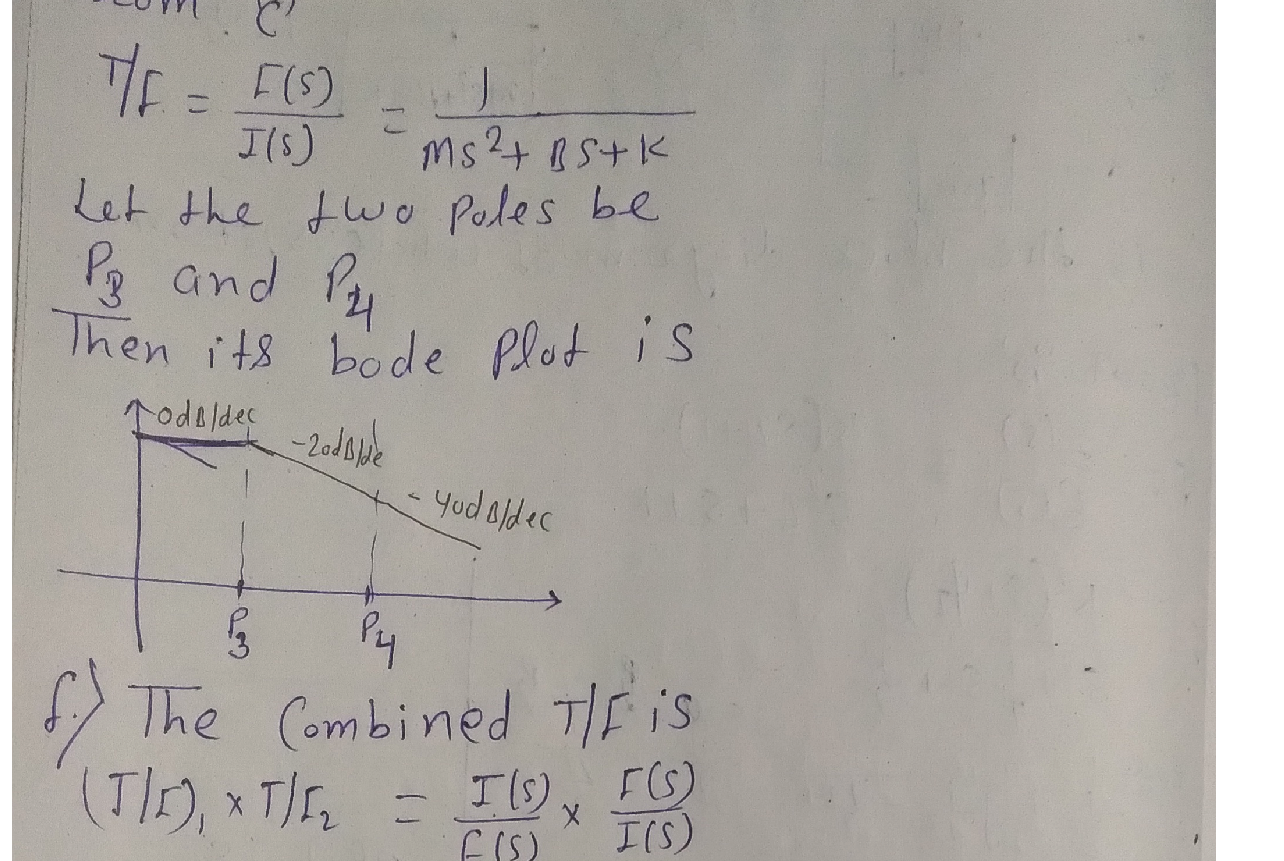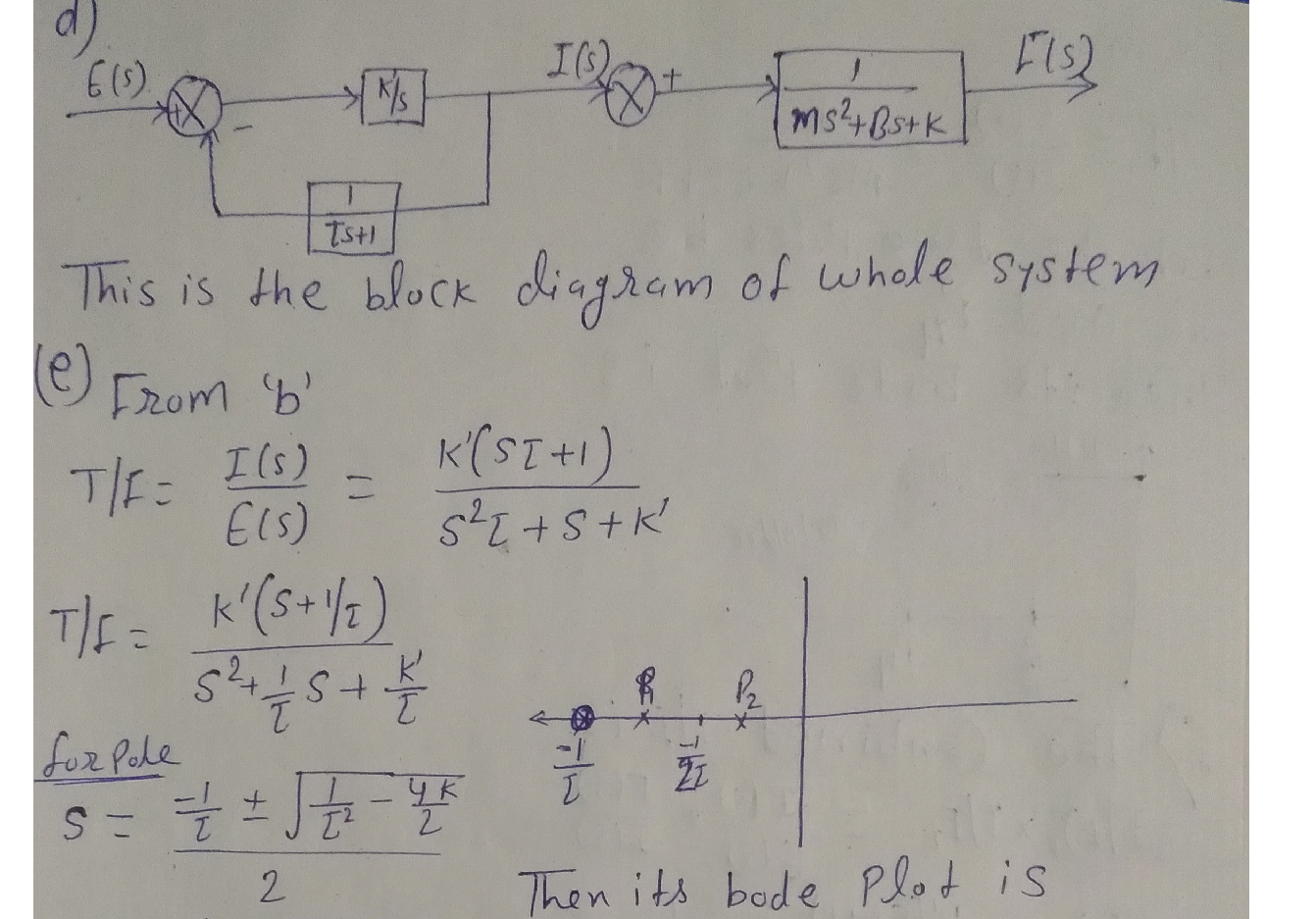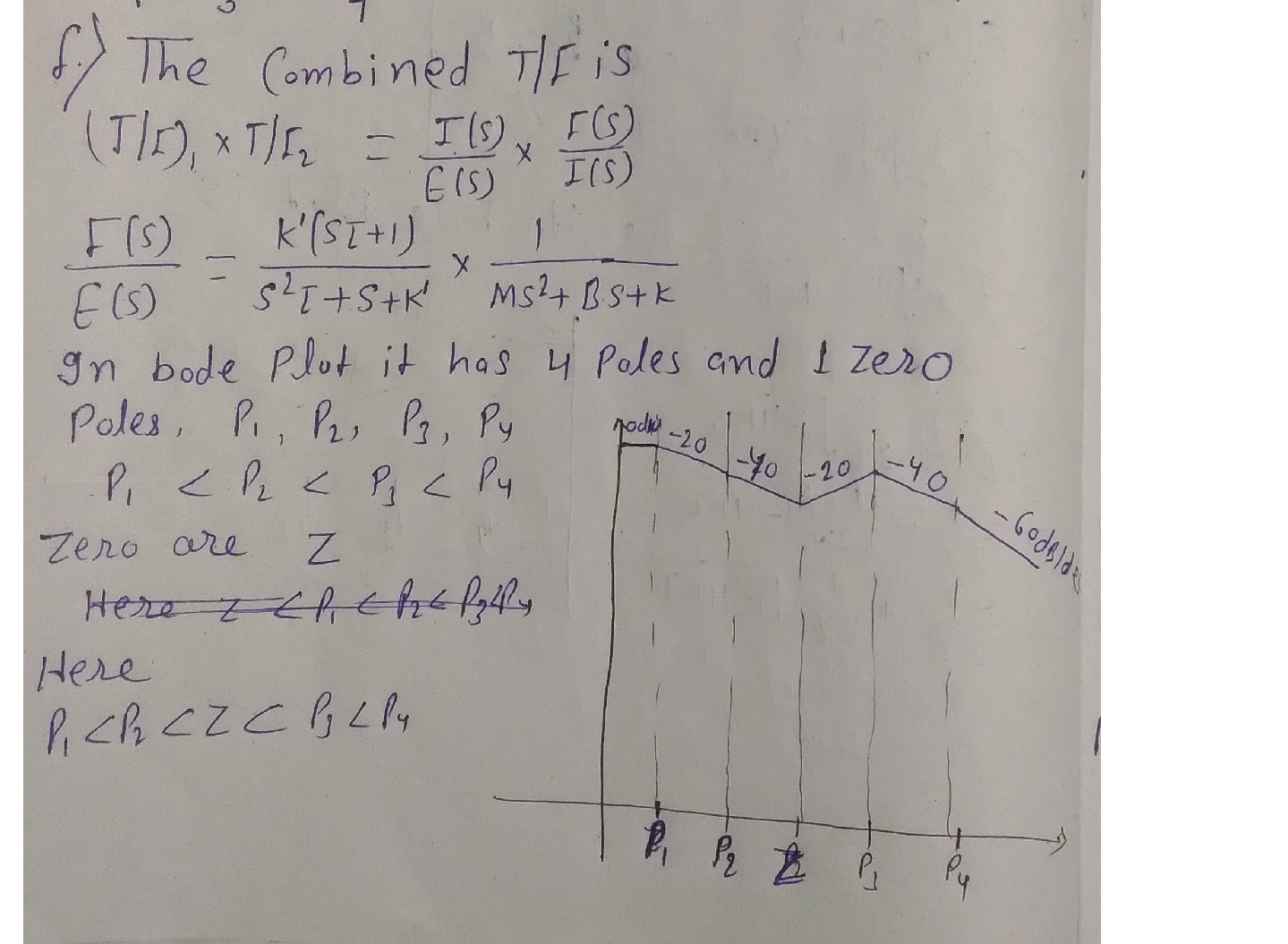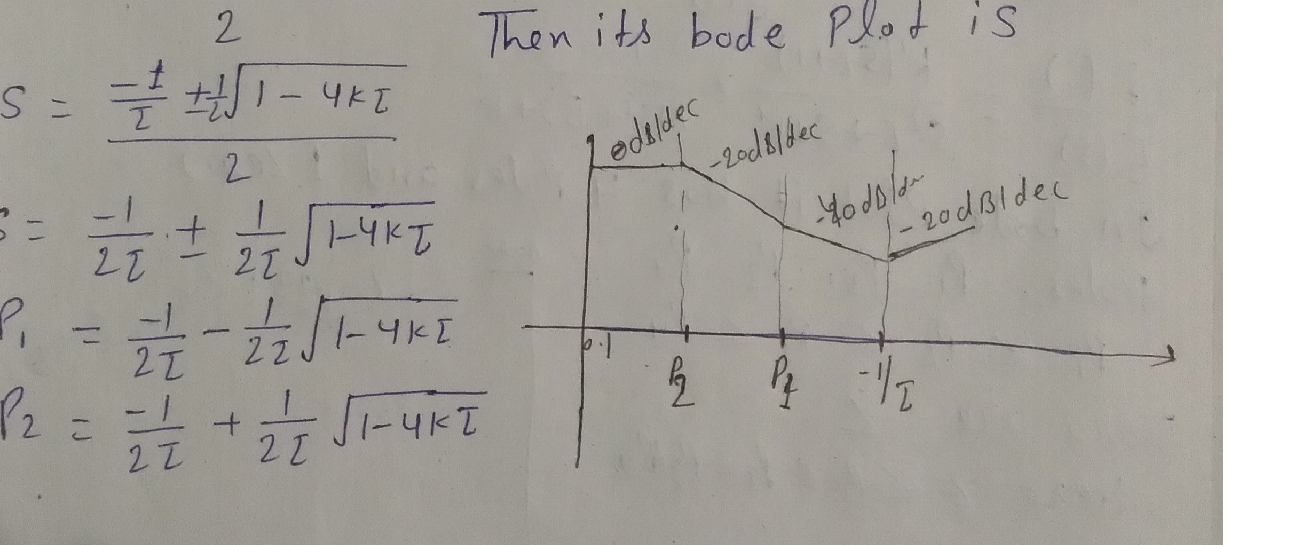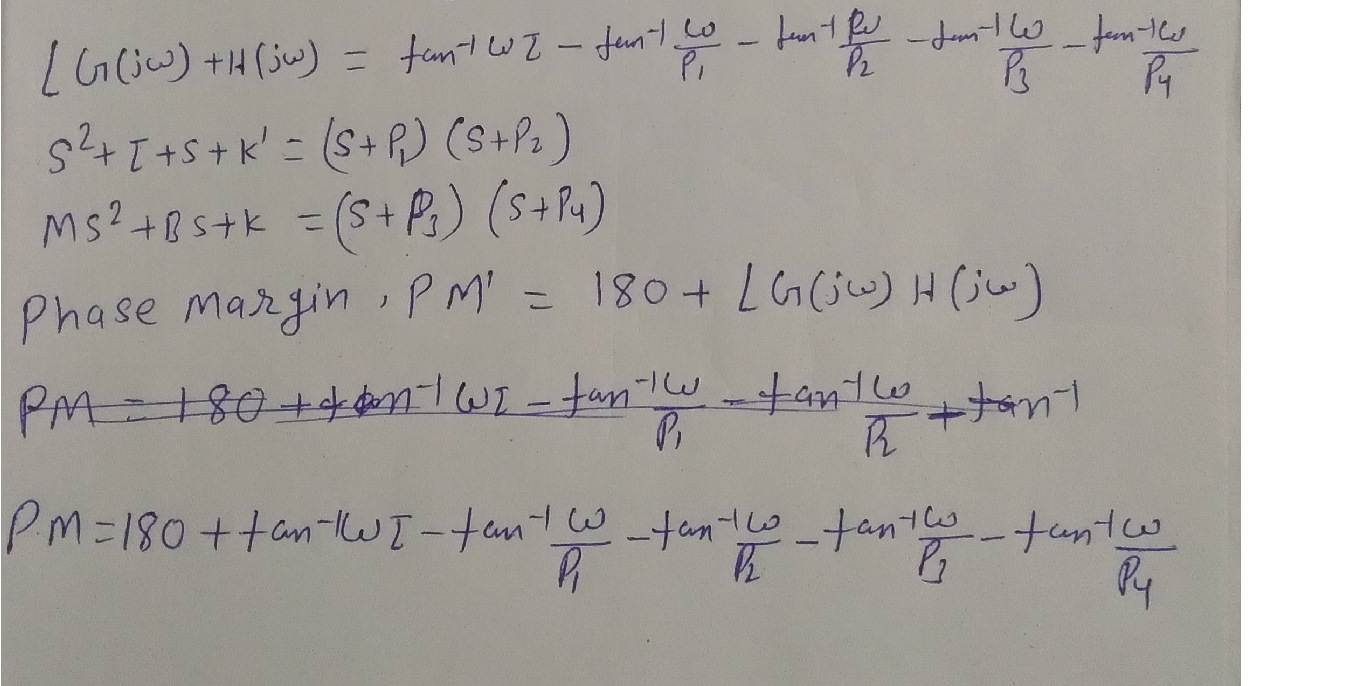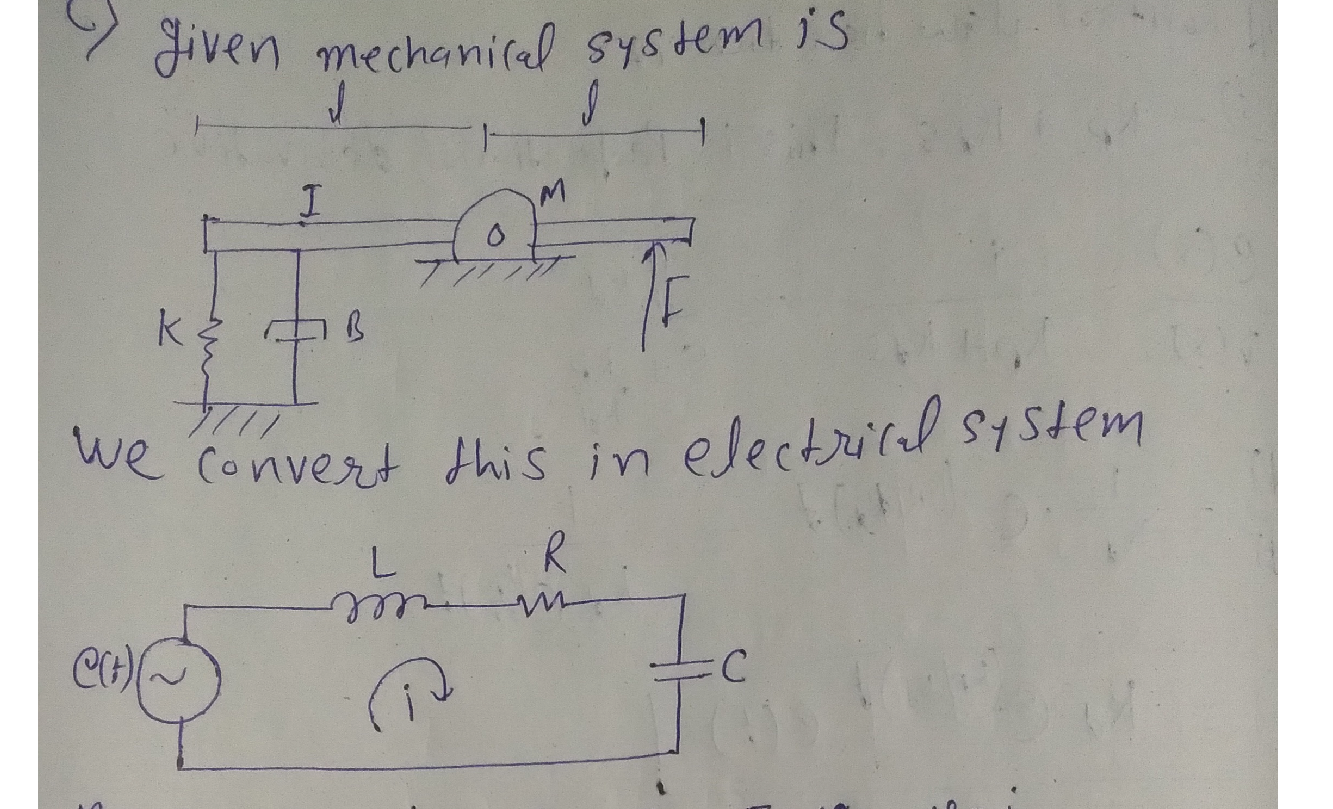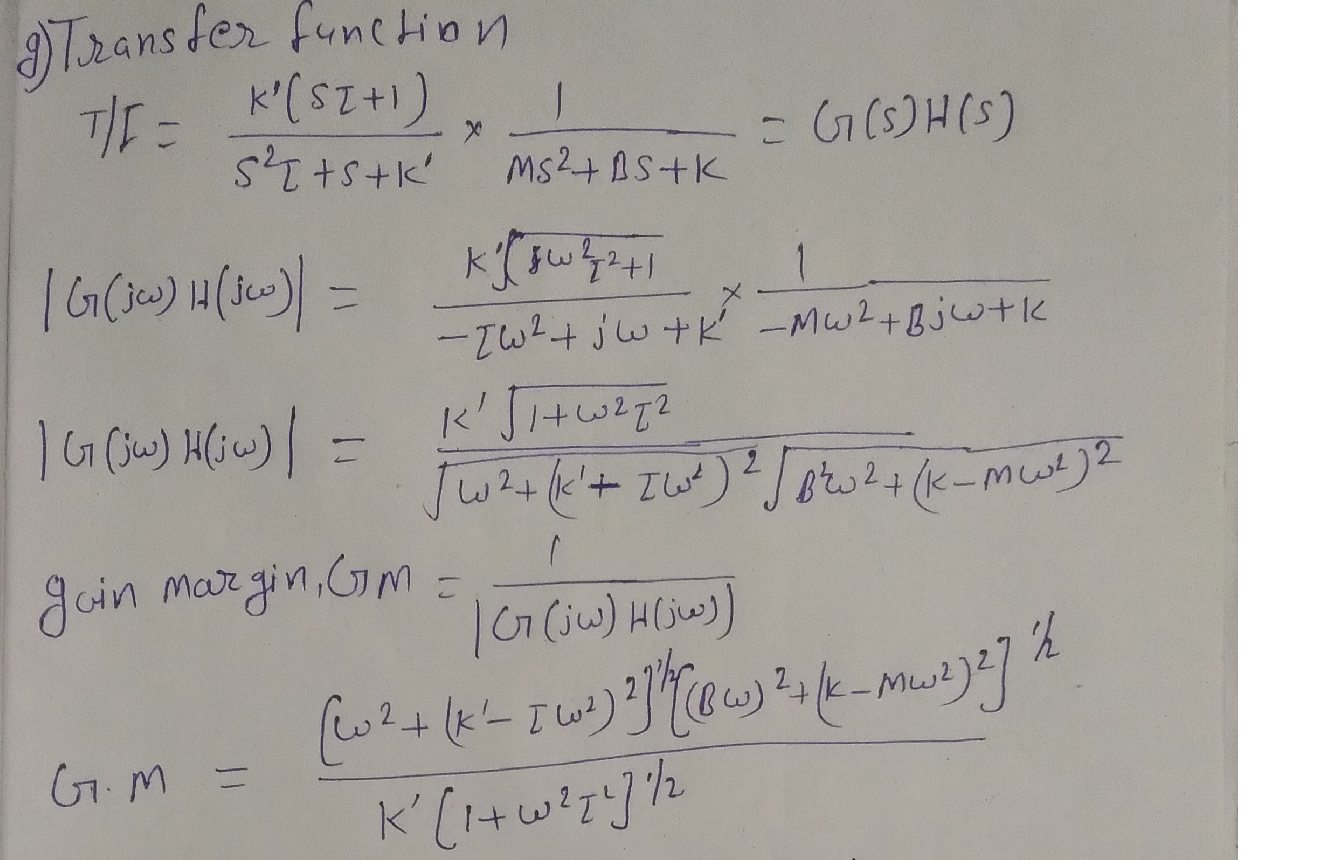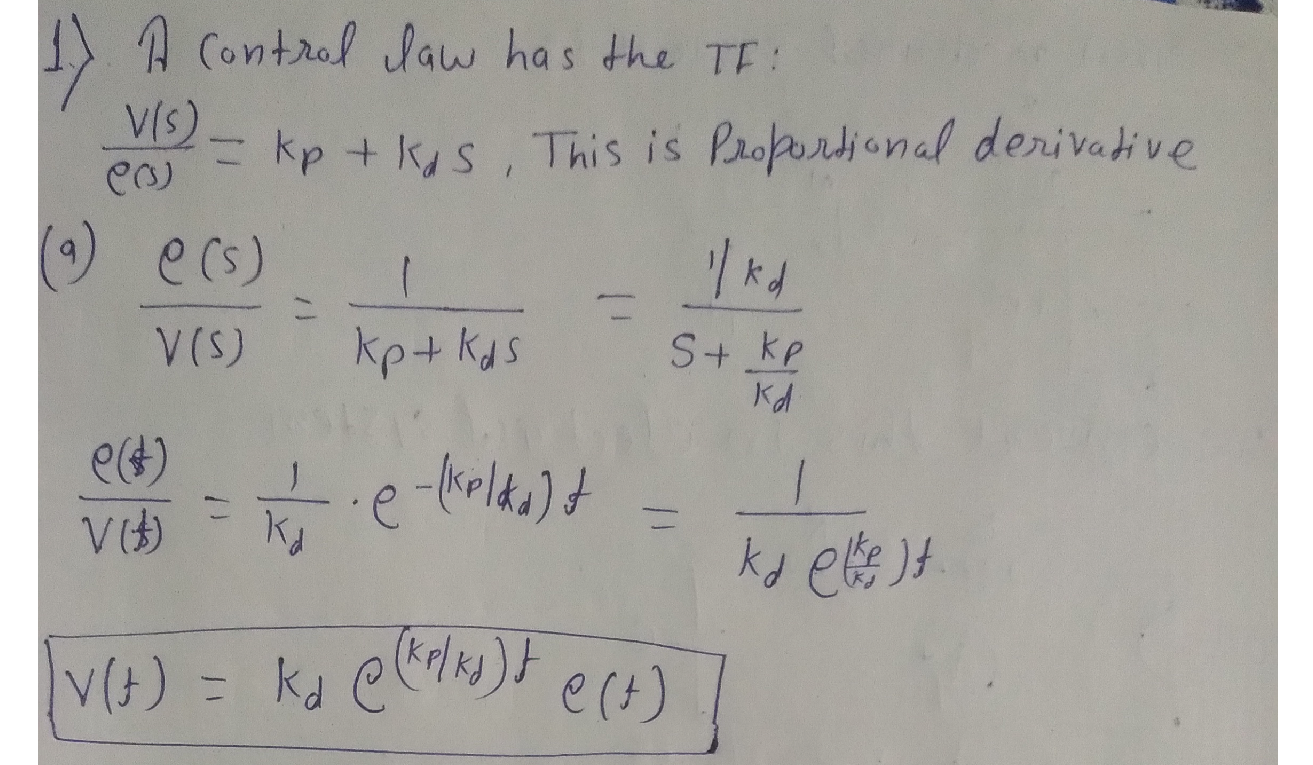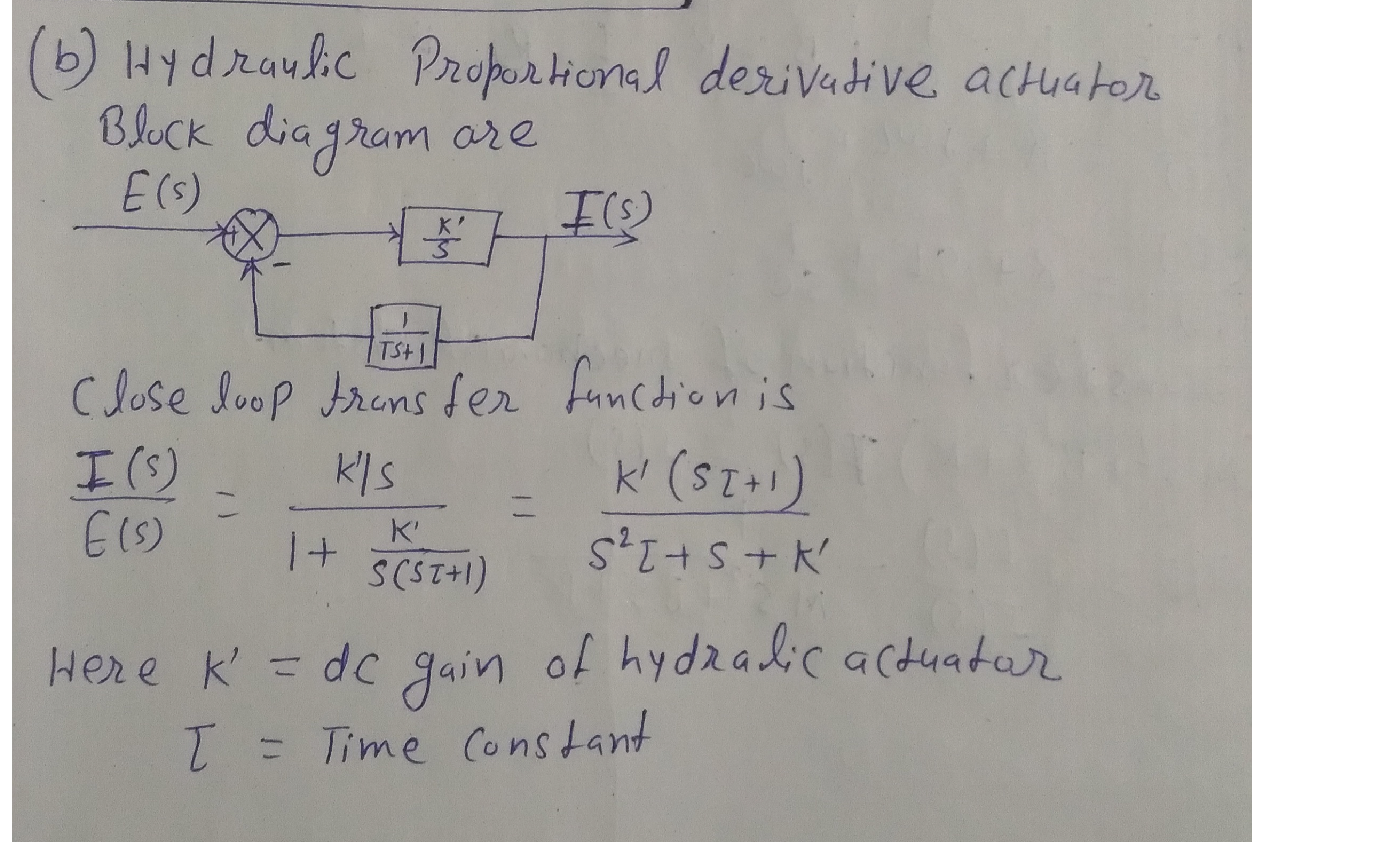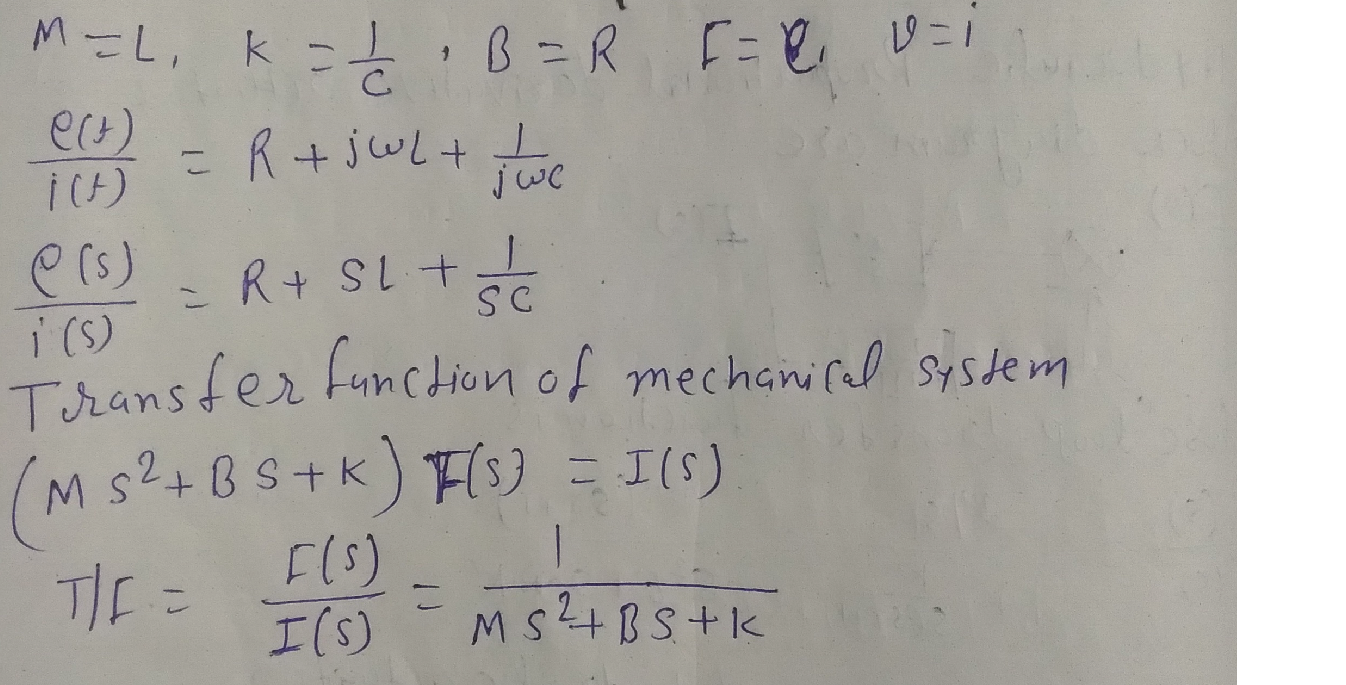### Question 45571Power Systems

State the advantages and disadvantages of using Time-of-Use (TOU) pricing strategy for EV charging.

### Question 45570Power Systems

Discuss the measures to mitigate EV load impacts by upgrading the distribution system infrastructure.

### Question 45569Power Systems

State the differences between North American distribution circuit and European distribution circuit.

### Question 45568Power Systems

Discuss the various impacts of electric vehicle load on the distribution system.

### Question 45567Power Systems

Discuss the mitigation measures for high voltage and low voltage impacts of Perpetration.

### Question 45566Power Systems

Enumerate the functions of a smart inverter.

### Question 45565Power Systems

Discuss the impact of high PV penetration on automatic voltage regulation equipment.

### Question 45564Power Systems

What is a masked load? How does it occur? Discuss its impact on the distribution circuits.

### Question 45563Power Systems

Enumerate the various impacts of high PV penetration on the distribution system.

### Question 45562Power Systems

How does the document define “high penetration PV"?

### Submit query